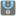• # The Most Numbers -> i Dont know how to solve last.

## Question related to missionThe Most Numbers

def checkio(args): absmax=abs(max(args)) absmin=abs(min(args)) if len(args)==0: return 0 elif max(args)<0 and min(*args)<0: return abs(absmax-absmin) elif max(*args)>0 and min(args)<0: return absmax+absmin elif max(*args)>0 and min(args)>0: return absmax-absmin else: return 0

# These "asserts" using only for self-checking and not necessary for auto-testing

if name == 'main': def almostequal(checked, correct, significantdigits): precision = 0.1 ** significant_digits return correct - precision < checked < correct + precision

```print('Example:')
print(checkio(1, 2, 3))

assert almost_equal(checkio(1, 2, 3), 2, 3), "3-1=2"
assert almost_equal(checkio(5, -5), 10, 3), "5-(-5)=10"
assert almost_equal(checkio(10.2, -2.2, 0, 1.1, 0.5), 12.4, 3), "10.2-(-2.2)=12.4"
assert almost_equal(checkio(), 0, 3), "Empty"
print("Coding complete? Click 'Check' to review your tests and earn cool rewards!")
```

from this code, i dont know exactly last one checkio 4th, if i have tuple that dont have index 0, (ex a=()) than, how can i handle this tupple is in if loop... please any body help me :>5